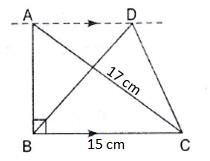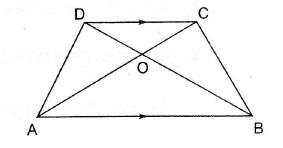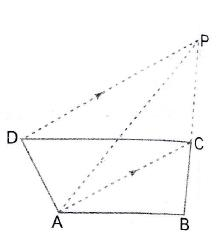Question 1: In the given figure,$\Delta ABC$ is right angled at$B$ in which$BC = 15 \ cm$ and$CA = 17 \ cm$. Find the area of acute angled$\Delta DBC$, it being given that$AD \parallel BC$$AB= \sqrt{(17^2- 15^2 )}=8\ cm$ (since$\Delta ABC$ is a right angled triangle)

Area of$\Delta DBC = \frac{1}{2} \times 15\times 8=60 \ cm^2$$\\$

Question 2: In the adjoining figure, area$(\parallel gm ABCD) = 48 cm^2 \ and\ FC \parallel AB \ \\ Find: \ i) \ Area \ of \parallel gm ABEF \ ii)\ Area \ of \Delta EAB$Area of$\parallel gm ABEF= Area \ of \parallel gm \ ABCD= 48 cm^2$

This is because$\parallel gm ABCD \ and\ \parallel gm \ ABEF$ are between the same parallels and have the same base.

Also, since$\Delta EAB \ and \ the \parallel gm \ ABCD$ are on the same base and between the same parallels, so the

Area of$\Delta EAB= \frac{1}{2} \times Area \ of \parallel gm \ ABCD=24 \ cm^2$$\\$

Question 3: In the adjoining figure, area$\Delta ABC = 27 cm^2 \ and\ EF \parallel BC.$ Find$: \ i) Area \ of \parallel gm \ ABCF \ ii) \ Area \ of rect. \ BCDE.$Also, since$\Delta ABC \ and \ the \parallel gm \ ABCF$ are on the same base and between the same parallels, so the

Area of$\Delta ABC= 1/2\times \ Area \ of \parallel gm \ ABCF$$\\Rightarrow Area \ of \parallel gm \ ABCF=2\times ( Area \ of \Delta ABC)=54cm^2$

Area of$\parallel gm \ BCDE= Area \ of \parallel gm ABCF= 54 cm^2$

This is because$\parallel gm \ ABCF \ and\ \parallel gm \ BCDE$ are between the same parallels and have the same base.$\\$

Question 4: In trapezium$ABCD$, it is being given that$AB \parallel DC$ and diagonals$AC \ and\ BD$ intersect at O. Prove that:$\ i) \ Area (\Delta DAB) =Area (\Delta CAB) \ ii) \ Area (\Delta AOD) =Area (\Delta BOC)$Since$\Delta EAB \ and\ \Delta CAB$ are on the same base and between the same parallels, the area of the two$\Delta$‘s will be equal.$\Rightarrow \ Area (\Delta DAB) =Area (\Delta CAB)$

Also Since$\Delta ACD \ and\ \Delta CBD$ are on the same base and between the same parallels,the area of the$\ two \Delta$‘s will be equal.$\Rightarrow \ Area (\Delta ACD)=Area (\Delta CBD)$

Now, subtract the area of$\Delta ODC$ from both sides we get$\Rightarrow \ Area (\Delta AOD) =Area (\Delta BOC)$$\\$

Question 5: In the adjoining figure,$ABCD$ is a parallelogram,$P$ is a point on$DC \ and \ Q \ is \ a \ point \ on \ BC$. Prove that :$i) \Delta APB \ and\ \Delta AQD$ are equal in area$ii) Area (\Delta AQD) = Area (\Delta ADP) + Area (\Delta BCP)$Since$\Delta APB \ and \ the \parallel gm ABCD$ are on the same base and between the same parallels, so the

Area of$\Delta APB= \frac{1}{2} \times \ Area \ of\ \parallel gm \ ABCD$

Similarly,$\Delta AQD \ and \ the \ \parallel gm \ ABCD$ are on the same base and between the same parallels, so the

Area of$\Delta AQD= \frac{1}{2} \times \ Area \ of \parallel gm \ ABCD$$\Rightarrow \Delta APB \ and\ \Delta AQD \ are \ equal \ in \ area$

Area$(\Delta ADP)+ Area (\Delta BCP)= \frac{1}{2} \times DP\times (height)+\frac{1}{2} \times PC\times (height)$$\Rightarrow Area (\Delta ADP)+ Area (\Delta BCP)= \frac{1}{2} \times (DP+PC)\times (height)$$= \frac{1}{2} \times (DC)\times (height)$$= \frac{1}{2} \times \ Area \ of\ \parallel gm \ ABCD = Area \ of \Delta AQD$

Hence Proved.$\\$

Question 6: In the adjoining figure,$ABCD$ is a quadrilateral. A line through$D$, parallel to$AC$, meets$BC$ produced in$P$. Prove that$Area (\Delta ABP) = Area (quad. ABCD)$.$Area (quad. ABCD)= Area (\Delta ABC)+ Area (\Delta ACD)$$Area (\Delta ACD)= \frac{1}{2} \times \ Area \ of \parallel gm \ ADPC$

Similary,$Area (\Delta PCD)= \frac{1}{2} \times \ Area \ of \parallel gm \ ADPC$$\Rightarrow Area (\Delta ACD)= Area (\Delta PCD)$

Hence$Area (quad. ABCD)= Area (\Delta ABC)+ Area (\Delta PCD)$

But$Area (\Delta PCD)= Area (\Delta PCA)$

Hence Proved that$Area (\Delta ABP) = Area (quad. ABCD)$$\\$

Question 7:$PQRS$ is any quadrilateral. Line segments passing through the vertices are drawn parallel to the diagonals of this quadrilateral so as to obtain a parallelogram$ABCD$ as shown in the adjoining figure. Prove that:$Area (quad. PQRS) = \frac{1}{2} \times \ Area (\parallel gm ABCD)$$Area (quad. PQRS)=Area (\Delta PQR)+ Area (\Delta PRS)$$= \frac{1}{2} \times \ Area \ of \parallel gm \ PRAB+ \frac{1}{2} \times \ Area \ of \parallel gm \ PRCD$
Since$\parallel gm \ ABCD= \parallel gm \ ABRP+ \parallel gm \ PRCD$$=\frac{1}{2} \times \ Area (\parallel gm \ ABCD)$